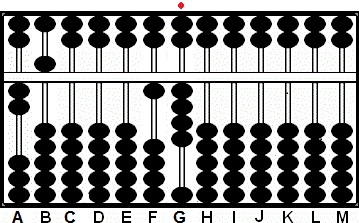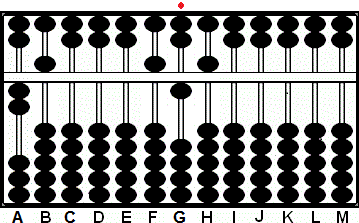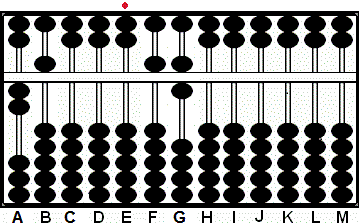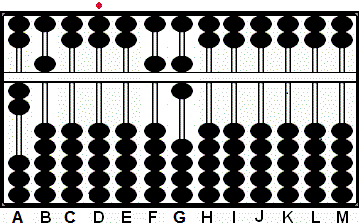###Suan Pan and the Unit Rod - Division

##### 1.003..... one whole number - unit rod shifts 1 rod to the left. 45.03..... two whole numbers - unit rod shifts 2 rods to the left...etc. 0.75....... no whole numbers, no trailing zeros, unit rod does *not* shift. 0.0125... one trailing zero - unit rod shifts 1 rod to the right. 0.0036... two trailing zeros - unit rod shifts 2 rods to the right...etc.

Using these Suan Pan techniques to determine a decimal answer is remarkably easy. In order to find the correct decimal answer one takes into into account either the whole numbers or the trailing zeros in the divisor and shifts the unit rod left or right as needed. To better illustrate this here are some decimal variations of the same problem. On a personal note I've always found using different decimal variants of the same problem to be a good way to get my head around working with decimals and unit rods.

Example 1: 14 ÷ 25 = 0.56

a) rule 1/2 = 5

Step 1: Choose rod G as the unit rod. Set divisor 25 on rods AB and 14 on rods FG. (Fig. 1)Fig. 1 ```Step 1  A B C D E F G H I J K L M       2 5 0 0 0 1 4 0 0 0 0 0 0 Step 1```

Step 2:  Compare 2 on A with 1 on rod F and follow ==> rule 1/2 = 5 <== The first number in the quotient will be will be 5. Place 5 on rod F.
Step 2a: Multiply 5 on F by 5 on B and subtract the product 25 from rods GH. This leaves the remainder 15 on rods GH. (Fig. 2)Fig. 2 ```Step 2  A B C D E F G H I J K L M       2 5 0 0 0 1 4 0 0 0 0 0 0 (5) Step 2 - 2 5           Step 2a 2 5 0 0 0 5 1 5 0 0 0 0 0 ```

Step 3:  Compare 2 on A with 1 on rod G and follow ==> rule 1/2 = 5 <== The second number in the quotient will be will be 5. Place 5 on rod G.
Step 3a: Multiply 5 on F by 5 on B and subtract the product 25 from rods HI. With 25 remaining on rods HI revise the quotient on G higher by 1.
Step 3b: Revise the quotient: add 1 to 5 on rod G and subtract a further 25 from rods HI. This leaves 56 on rods FG. (Fig. 3)Fig. 3 ```Step 3  A B C D E F G H I J K L M       2 5 0 0 0 5 1 5 0 0 0 0 0 (5) Step 3   - 2 5         Step 3a 2 5 0 0 0 5 5 2 5 0 0 0 0 Revise + 1 Step 3b   - 2 5         2 5 0 0 0 5 6 0 0 0 0 0 0 ```

Step 4 and the answer: Identify the new unit rod. Because the divisor 25 has two whole numbers shift the unit rod two to the left. The unit rod has now shifted to rod E leaving the answer. 0.56 on rods E - G. (Fig. 4)Fig. 4 ```Step 3  A B C D E F G H I J K L M 2 5 0 0 0 5 6 0 0 0 0 0 0 Step 4 ```

Decimal Variations

This next problem can be done in exactly in the same way but using different decimal numbers. The only thing that changes is the position of the unit rod.

Example 2: 0.014 ÷ 0.0025 = 5.6

Choose rod D to be the unit rod. Set divisor 25 on rods AB and 14 on rods FG (Fig. 1)Fig. 1 ```Step 1  A B C D E F G H I J K L M       2 5 0 0 0 1 4 0 0 0 0 0 0```

Continue with the problem exactly as above eventually arriving at the same answer. The only difference is the position of the unit rod is on rod D. (Fig. 2)Fig. 2 ```Step 2  A B C D E F G H I J K L M       2 5 0 0 0 5 6 0 0 0 0 0 0```

The final step and the answer: Identify the new unit rod. Because the divisor 0.0025 has two trailing zeros shift the unit rod two to the right. This leaves the answer 5.6 on rods FG. (Fig.3)Fig. 3 ```Step 3  A B C D E F G H I J K L M       2 5 0 0 0 5 6 0 0 0 0 0 0```

Other Examples

1.4 ÷ 25 = 0.056
140 ÷ 0.25 = 560
14 ÷ 0.0025 = 5,600
0.0014 ÷ 25 = 0.000056  .... etc. etc.